# RD Sharma Class 8 Solutions Chapter 3 Squares and Square Roots Ex 3.8

In this chapter, we provide RD Sharma Class 8 Solutions Chapter 3 Squares and Square Roots Ex 3.8 for English medium students, Which will very helpful for every student in their exams. Students can download the latest RD Sharma Class 8 Solutions Chapter 3 Squares and Square Roots Ex 3.8 Maths pdf, free RD Sharma Class 8 Solutions Chapter 3 Squares and Square Roots Ex 3.8 Maths book pdf download. Now you will get step by step solution to each question.

### RD Sharma Solutions for Class 8 Chapter 3 Squares and Square Roots Ex 3.8 Download PDF

Question 1.
Find the square root of each of the following correct to three places of decimal :
(i) 5
(ii) 7
(iii) 17
(iv) 20
(v) 66
(vi) 427
(vii) 1.7
(vii’) 23.1
(ix) 2.5
(x) 237.615
(xi) 15.3215
(xii) 0.9
(xiii) 0.1
(xiv) 0.016
(xv) 0.00064
(xvi) 0.019
(xvii) 78
(xviii) 512
(xix) 2 12
(xx) 287 88
Solution: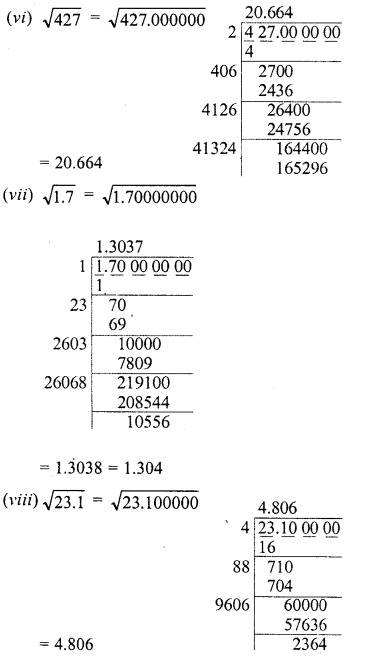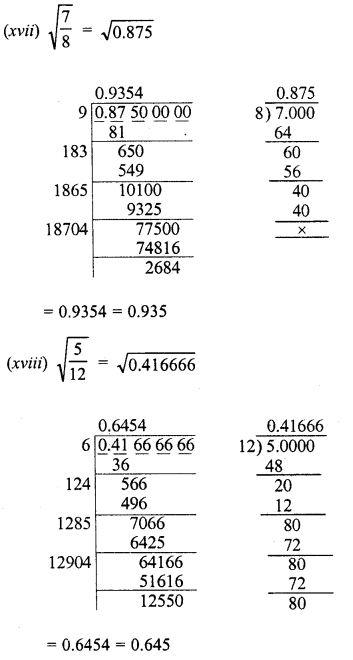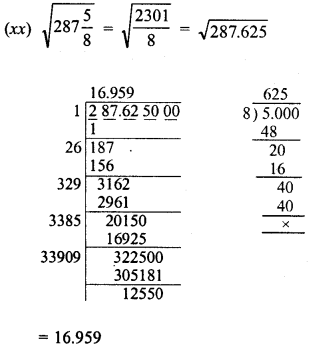Question 2.
Find the square root of 12.0068 correct to four decimal places.
Solution:Question 3.
Find the square root of 11 correct to five decimal places.
Solution: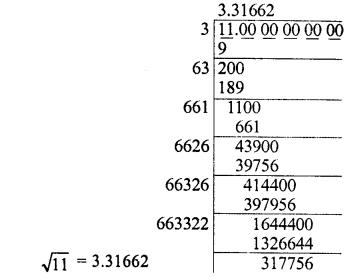Question 4.
Given that √2, = 1-414, √3 = 1.732, √5 = 2.236 and √7 = 2.646. Evaluate each of the following :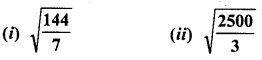Solution: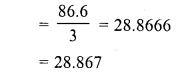Question 5.
Given that √2 = 1-414, √3 = 1-732, √5= 2.236 and √7= 2.646, find the square roots of the following :Solution: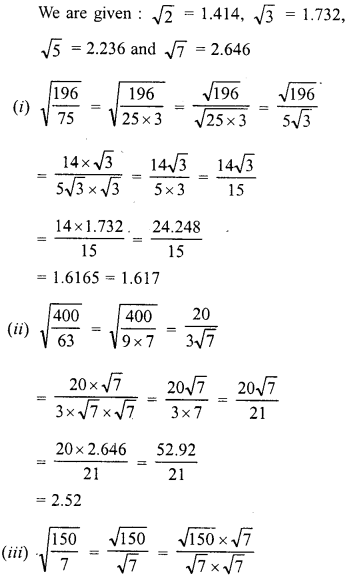All Chapter RD Sharma Solutions For Class 8 Maths

—————————————————————————–

All Subject NCERT Exemplar Problems Solutions For Class 8

All Subject NCERT Solutions For Class 8

*************************************************

I think you got complete solutions for this chapter. If You have any queries regarding this chapter, please comment on the below section our subject teacher will answer you. We tried our best to give complete solutions so you got good marks in your exam.

If these solutions have helped you, you can also share rdsharmasolutions.in to your friends.